multi-objective programming

V.帕雷托

• matlab程序 多目标规划_ 多目标规划_ 多目标规划_
• 文档主要介绍了多目标规划问题的相关模型的建立与求解，有详细实例。
• 对于多于一个的目标函数在给定区域上的最优化问题称为多目标规划问题。在多目标规划中，各目标之间是相互冲突的，不一定存在所有目标上都是最优的解。因此多目标问题的解构成一个集合，他们之间不能简单地比较好坏，...
author：旭宝ww
DateTime：2020/7/2

一、引言
对于多于一个的目标函数在给定区域上的最优化问题称为多目标规划问题。在多目标规划中，各目标之间是相互冲突的，不一定存在所有目标上都是最优的解。因此多目标问题的解构成一个集合，他们之间不能简单地比较好坏，这样的解称为非支配解（有效解） 或者 Pareto最优解。
注意：多目标规划不同于单目标规划，在数学建模的结果中不应当给出一个最优解，Pareto最优解应当是一组解，以供甲方选择。
二、模型实例
1 一般地，多目标规划的数学模型如下：

$\mathbf{min \quad F(x)=\left (f_1(x),f_2(x),…,f_m(x)\right) }\\ \mathbf{s.t.\quad x\in \Omega}$

其中$x=(x_1,x_2,…,x_n)$所在的空间$\Omega$称为问题的决策空间（可行解空间），m维向量$F(x)$所在的空间称为问题的目标空间。
其中$\Omega =\left \{x\in R^{n} |g_i(x)\le 0,i=1,2,…,p \right \}$
2 相关概念
定义1：对最小化问题，一个向量$u=(u_1,u_2,…,u_m)$支配另一个向量$v=(v_1,v_2,…,v_m)$，当且仅当
$\begin{cases} u_i \le v_i,\forall i \in \left \{ 1,2,…，m \right \} \\ \exists j \in \left \{ 1,2,…,m \right \} \end{cases}$
定义2：对于任意两个自变量向量$x_1,x_2\in \Omega$，如果向量$(f_1(x_1),f_2(x_1),…,f_m(x_1))$支配向量$(f_1(x_2),f_2(x_2),…,f_m(x_2))$，则称$x_1$支配$x_2$。
定义3：如果$\Omega$中没有支配$x$的解，则称$x$是问题的一个Pareto最优解，Pareto最优解的集合称为Pareto最优解集，Pareto最优解集在目标空间的像集称为Pareto前沿（Pareto Front）。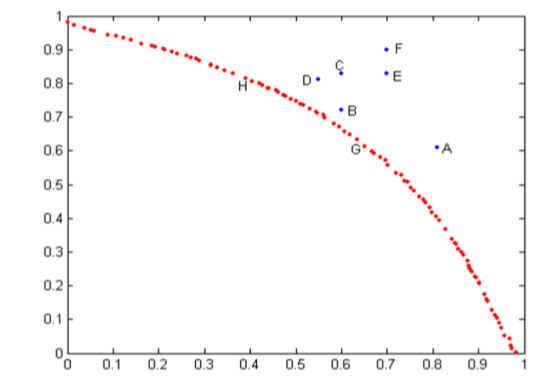例如，此图的横坐标为$F_1(x)$，纵坐标为$F_2(x)$，红色的连线即为该问题的Pareto前沿，H和G两点的自变量$x$都是此问题的解。
三、求解方法
多目标优化问题的算法大体分为传统的数学规划方法和智能优化方法。传统方法只能得到非支配解，故需要重点掌握智能优化方法的MATLAB实现。
3.1传统的数学规划方法
1.主要目标法：确定一个目标为主要目标，确定合适的界限值把次要目标作为约束条件。
$\begin{cases} min \quad f_1(x)\\ s.t. \quad f_i(x) \le a_i,i=2,3,…,m\\ \quad\quad x \in \Omega \end{cases}$
其中界限值$a_i\ge \min \limits_{x\in \Omega} f_i(x),i=2,3,…,m$
2.分层序列法：先对m个目标的重要性排序。先求问题：
$P(1)\quad \begin{cases} min \quad f_1(x)\\ s.t.\quad x \in \Omega \end{cases}$
的最优解$x^{(1)}$和最优值$f_1^{*}$。
再求问题：
$P(2)\quad \begin{cases} min \quad f_2(x)\\ s.t.\quad x \in \Omega_1=\Omega \bigcap \left \{ x|f_1(x) \le f_1^* \right \} \end{cases}$
的最优解$x^{(2)}$和最优值$f_2^{*}$。
如此进行下去，直到求出第$m$个问题:
$P(m)\quad \begin{cases} min \quad f_m(x)\\ s.t.\quad x \in \Omega_{m-1}=\Omega_{m-2} \bigcap \left \{ x|f_{m-1}(x) \le f_{m-1}^* \right \} \end{cases}$
的最优解$x^{(m)}$和最优值$f_m^{*}$。
于是$x^*=x^{(m)}$就是多目标规划问题的一个非支配解。
3.加权法：对m个目标加以适当的权重，化作单目标规划
$\begin{cases} min \sum\limits_{i=1}^{m}\omega_if_i(x)\\ s.t.\quad x \in \Omega \end{cases}$
4.MATLAB求解单目标规划问题

[x,fval]=quadprog(H,f,A,b,Aeq,beq,lb,ub)                  %%求解二次规划$x^TAx$
[x,fval]=fmincon(f,x0,A,b,Aeq,beq,lb,ub,nonlcon)    %%求解约束下的多元函数最小值

3.2 智能优化算法（NSGA-Ⅱ）
能力有限，无法理解算法的原理，贴一位大佬的博客：
https://blog.csdn.net/qq_40434430/article/details/82876572
MATLAB中的内置函数gamultiobj() （ga:遗传；multiobj:多目标 ）是基于NSGA-Ⅱ改进的一种多目标遗传算法。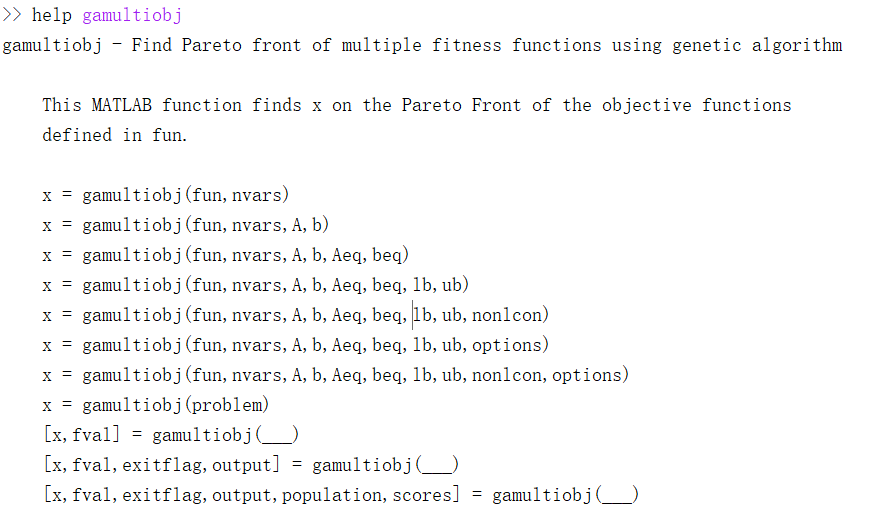gamultiobj适用于求解以下形式的多目标问题：
$\begin{cases} min \quad F(x)\\ s.t.\quad AX\le b\\ \qquad Aeq*X=beq\\ \qquad lb\le X\le ub \end{cases}$
调用格式：

[X,FVAL]=gamultiobj( f, NVARS, A, b, Aeq, beq, lb, ub, nonlcon, options)
%X是Pareto解集
%FVAL是目标函数在Pareto解上的函数值
%f是需要计算的目标函数
%NVARS是变量的个数
%options是绘制Pareto前沿的函数gaoptimset的参数

实例：
$\begin{cases} min\quad f_1(x)=x_1^4-10x_1^2+x_1x_2+x_2^4-x_1^2x_2^2\\ min\quad f_2(x)=x_1^4+x_1x_2+x_2^4-x_1^2x_2^2\\ s.t.\quad -5\le x_1,x_2\le 5 \end{cases}$
function y=Fun(x)
y(1)=x(1)^4-10*x(1)^2+x(1)*x(2)+x(2)^4-x(1)^2*x(2)^2;
y(2)=x(2)^4-x(1)^2*x(2)^2+x(1)^4+x(1)*x(2);
end

clear;clc;
fitnessfcn=@Fun	%%调用函数句柄
nvars=2; %%变量个数
lb=[-5,-5];
ub=[5,5];
A=[];b=[];
Aeq=[];beq=[];
options=gaoptimset('paretoFraction',0.3,'populationsize',100,'generations',200,'stallGenLimit',200,'TolFun',1e-10,'PlotFcns',@gaplotpareto);
%最优个体系数paretoFraction为0.3
%种群大小populationsize为100
%最大进化代数generations为200
%停止代数stallGenLimit为200
%适应度函数偏差TolFun为1e-10
%函数gaplotpareto：绘制Pareto前沿
[x,fval]=gamultiobj(fitnessfcn,nvars,A,b,Aeq,beq,lb,ub,options)

MATLAB运行结果如下：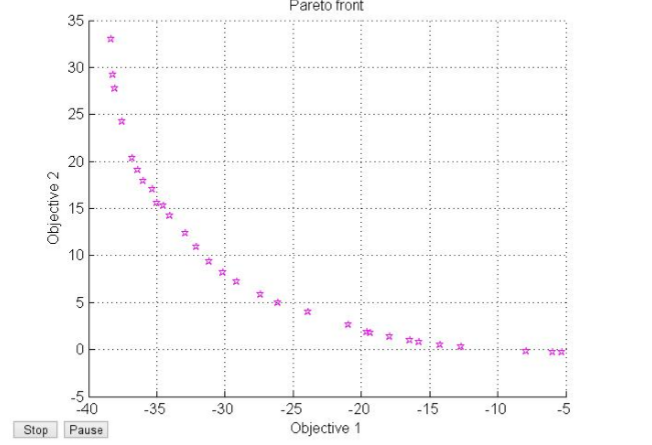展开全文matlab 数学建模
• 多目标规划模型多目标规划模型的基本知识多目标规划模型的绝对最优解多目标规划模型的有效解多目标规划模型的弱有效解多目标规划模型的常用解法介绍1. 主要目标法2. 分层序列法3．线性加权求和法多目标规划建模示例1...
多目标规划模型多目标规划模型的基本知识多目标规划模型的绝对最优解多目标规划模型的有效解多目标规划模型的弱有效解多目标规划模型的常用解法介绍1.  主要目标法2.  分层序列法3．线性加权求和法多目标规划建模示例1. 模型假设2. 模型建立3. 模型求解
多目标规划模型的基本知识
前面介绍的线性规划模型、整数规划模型、非线性规划模型中的目标函数都只有一个（可以进我的博客查看详细）。而在许多实际问题中，衡量一个方案好坏的标准往往不止一个，例如设计导弹，既要射程最远，又要燃料最省，还要精度最高。这一类问题称为多目标规划问题。对多目标规划问题建立其具有多个目标函数的数学规划模型称为多目标规划模型。
具有个目标的多目标规划模型的一般形式为：
目标函数：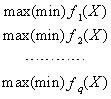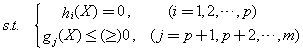由于等式约束总可以转化为不等式约束，大于等于约束总可以转化为小于等于约束，同时目标函数的最大化总可以转化为目标函数的最小化，于是多目标规划模型的一般形式又可简化为：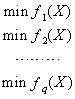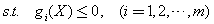称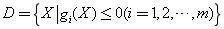为多目标规划模型的可行域。多目标规划模型的一般形式又可表达为：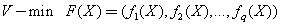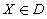其中V-min表示对个目标f1(X)，f2(X)，…，fq(X)以追求最小为目的。
设有两向量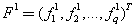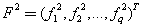规定以下几个有关向量比较的符号：
（1）符号“≤”：若 F1≤F2，则意味着F1的每个分量都要小于或等于F2的对应的分量；
（2）符号“<”：若F1<F2，则意味着F1的每个分量都要严格小于F2的对应的分量；
（3）符号“≤”：若 F1≤F2，则意味着F1的每个分量都要小于或等于F2的对应的分量，并且存在F1的某一个分量严格的小于F2的对应的分量。
多目标规划模型的绝对最优解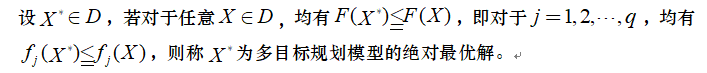多目标规划模型的有效解多目标规划模型的弱有效解在多目标规划模型的个目标中，有的相互联系，有的相互制约，有的相互冲突。多目标规划模型除了目标函数不只一个这一明显的特点外，最显著的还有以下两点：目标间的不可公度性和目标间的矛盾性。

目标间的不可公度性
目标间的不可公度性是指各个目标没有统一的度量标准，因而难以直接进行比较。例如房屋设计问题中，造价目标的单位是元/平方米，建造时间目标的单位是年，而结构、造型等目标则为定性指标；

目标间的矛盾性
目标间的矛盾性是指如果选择一种方案以改进某一目标的值，可能会使另一目标的值变坏，如房屋设计中造型、抗震性能目标的提高可能会使房屋建造成本目标提高。正是由于目标间的矛盾性，解决实际问题所建立的多目标规划模型常常没有绝对最优解，只能寻找其有效解或弱有效解。

多目标规划模型的常用解法介绍
多目标规划模型的解法大致可分为两类：直接解法和间接解法。到目前为止，常用的多为间接解法，即根据问题的实际背景和特征，设法将多目标规划模型转化为单目标规划模型，从而得到多目标规划模型的满意解。
1.  主要目标法
在多目标规划模型中，若能从q个目标中，确定一个目标为主要目标，例如f1(X)，而把其余目标作为次要目标，并根据实际情况，确定适当的界限值，这样就可以把次要目标作为约束来处理，而将多目标规划模型转化为求解如下的单目标线性或非线性规划模型：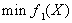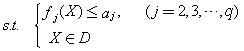其中界限值取为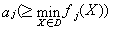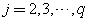则此单目标规划模型的最优解必为原多目标规划模型的弱有效解。因此，用主要目标法求得的解必是多目标规划模型的弱有效解或有效解。
2.  分层序列法
把多目标规划模型中的q个目标按其重要程度排一个次序，假设f1(X)最重要，f2(X)次之 ，f3(X)再次之，… ，最后一个目标为fq(X)。
先求出以第一个目标f1(X)为目标函数，而原模型中的约束条件不变的问题P1：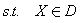其最优解为X1，最优值为f1*。再求解问题P2：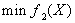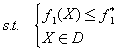其最优解为X2，最优值为f2*。再求解问题P3：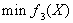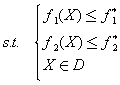其最优解为X3，最优值为f3*，… ，如此继续下去，直到求解第q个问题Pq：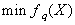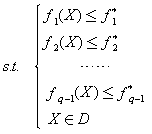其最优解为Xq，最优值为fq*。则X*=Xq就是原多目标规划模型在分层序列意义下得最优解，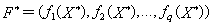为其最优值。
常将分层序列法修改如下：选取一组适当小的正数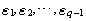成为宽容值，把上述的问题Pj修改如下：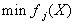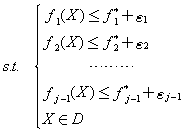再按上述方法依次求解各问题P1,P2,…,Pq 。
3．线性加权求和法
对多目标规划模型中的q个目标按其重要程度给以适当的非负权系数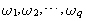且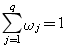，然后用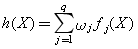作为新的目标函数，成为评价（目标）函数，再求解单目标规划问题：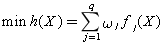多目标规划建模示例

选课策略问题

某学校规定，运筹学专业的学生毕业时必须至少学习两门数学课程、三门运筹学课程和两门计算机课程。这些课程的编号、名称 、学分、所属类别和先修课程要求如表所示。一般学生选课时要考虑总的课程门数和所获得的学分。试设计选课策略，在满足毕业要求的同时，使得所修课程门数尽量少，所获得的学分尽量多。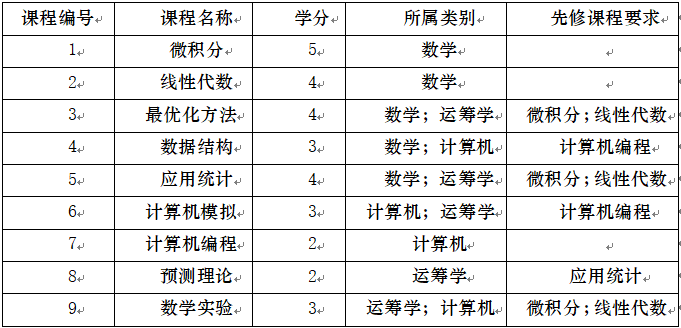1. 模型假设
（1）各个同学在选课时不受其他因素影响，只受学分和选课门数影响；
（2）各门课程没有人数限制；
（3）仅考虑表所列9门课程。
2. 模型建立
（1）决策变量
定义如下0-1变量作为决策变量：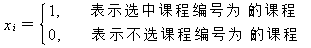（2）约束条件
至少选两门数学课程、三门运筹学课程和两门计算机课程，根据表中对各门课程所属类别的划分，这一约束可以表示为：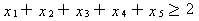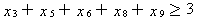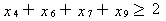某些课程有先修课程的要求。例如“数据结构”的先修课是“计算机编程”，这意味着如果x4=1，必须x7=1，这个条件可以表示为x4≤x7（注意：x4=0时对x7没有限制）。“最优化方法”的先修课是“微积分”和“线性代数”这一条件可以表示为x3≤x1，x3≤x2，而这两个不等式可以合并为2x3-x1-x2≤0。这样，所有课程的先修课要求可以表达为：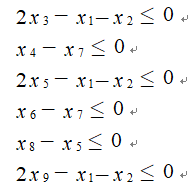（3）目标函数
根据本问题的假设，我们考虑课程门数和学分两个目标。
所选课程门数可表达为：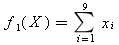我们希望课程门数尽量少，即希望对目标函数f1(X)实现最小化。
所选课程的总学分可表达为：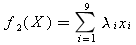其中λi为课程编号为i的课程的学分，这里λ1=5,λ2=4,λ3=4,λ4=3,λ5=4,λ6=3,λ7=2,λ8=2,λ9=3。
我们希望学分尽量多，即希望对目标函数f2(X)实现最大化。
综合上述分析，选课策略问题的数学模型为如下多目标规划模型：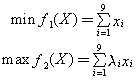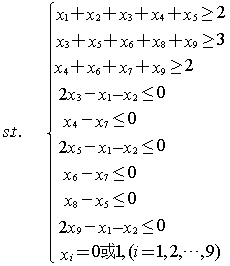3. 模型求解
记上述多目标规划模型的可行域为D。
（1）如果以课程门数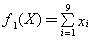作为主要目标，不考虑学分的多少，由主要目标法将上述多目标规划模型转化为如下的单目标规划模型：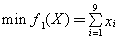运用LINGO软件求解得到：
x1=x2=x3=x6=x7=x9=1;    x4=x5=x8=0
即选微积分、线性代数、最优化方法、计算机模拟、计算机编程、数学实验这6门课，可满足毕业要求并使课程门数最少，此时总学分为21。
（2）如果以课程门数作为最重要目标，总学分作为次重要目标，即在课程门数达到最少的前提下使总学分达到最多。于是利用分层序列法在上述的基础上，再次求解如下的单目标规划模型：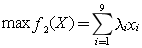运用LINGO软件求解得到：
x1=x2=x3=x5=x7=x9=1;    x4=x6=x8=0
即将上述（1）中学分的“计算机模拟”换成4学分的“应用统计”，同样这6门课，可使总学分由21增至22。
（3）如果认为课程门数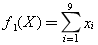和总学分这两个目标的重要程度之间的差异不十分明显，这时可由线性加权求和法取权系数0.7和0.3，将原多目标规划模型转化为如下的单目标规划模型：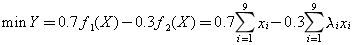运用LINGO软件求解得到：
x1=x2=x3=x4=x5=x6=x7=x9=1;    x8=0
即选微积分、线性代数、最优化方法、数据结构、应用统计、计算机模拟、计算机编程、数学实验这8门课。此时虽然课程门数增加了两门课，但总学分也增加到了28。
有用的话希望小伙伴们来个三连击，这是对我最大的鼓励
也让更多的人能够看到这篇文章！！！
帅气、靓丽的来个赞和关注
帅气、靓丽的来个赞和关注
帅气、靓丽的来个赞和关注


展开全文算法 matlab
• 多目标规划的几个充分性条件，杭丹，王晓燕，对于带不等式和等式约束的一般多目标规划问题，对其最有性条件进行了讨论，在较弱的条件下，对既带不等式约束又带等式约束的多目
• 以年均收益率和配电系统电压分布改善率最大化为目标构建多场景分布式电源多目标规划模型，并采用基于HAC种群截断策略的改进非劣排序复合微分进化算法对模型进行求解；以IEEE33节点配电系统为例进行了分布式电源...
• MATLAB求解多目标规划;一0-1规划的MATLAB求解;数学模型MIN lambda S.T. F(x)-weight* lambda (达到目标 Ax线性不等式约束 Aeqx=beq线性等式约束 C(x非线性不等式约束 Ceq(x)=0非线性等式约束 lb[f1(x,f2(x,]为多目标...
• 建立实际问题的多目标规划数学模型并求解是运筹学中常遇到的问题,应用最优化软件LINGO可以快捷准确地求出该类问题的解，本文以实例的方式介绍了多目标规划数学模型的建立、LINGO求解程序的编写，为实际工作者解决这...
• 分布式电源并网的多目标规划与决策研究，熊兰，吴雄，针对DG选址定容问题，建立了综合网损、电压偏差和电压稳定性的多目标模型，并提出了一种改进多目标差分进化算法。在传统多目标差�
• 数学思维之多目标规划，提升思维逻辑，建立模型方法，从模型建立到解法，样样俱全，包学包会，不学不会。没有一点数学基础的就算了吧，不适合太白金星。
• 27. 多目标规划 一线性规划的局限性 1. 线性规划要求所求解问题必须满足全部的约束而实际问题中并非所有约束都需要严格的满足 2. 线性规划只能处理单目标的优化问题从而对一些次目标只能转化为约束处理而实际问题中...
• 以最大化年寿命周期收益率和电压分布改善率作为目标函数，建立分布式电源多目标规划模型，并采用多目标复合微分进化算法对其求解和基于最短归一化距离法实现多目标总体最优解决策。以IEEE 33节点配电系统为例进行...
• 多目标规划在深圳市某电子公司决策中的应用致力于为大家提供学习、参考最实用的资源，对多目标规划在深圳...该文档为多目标规划在深圳市某电子公司决策中的应用，是一份很不错的参考资料，具有较高参考价值，感兴趣...
• 三级供应链应急转运策略优化的多层多目标规划模型，许长延，汪传旭，针对由一个工厂、多个货物集散中心和多个零售商组成的三级供应链系统，考虑零售商销售多种产品并采取应急转运策略。建立零售商订
• 鉴于此,首先基于区间多目标规划建立一个以预期收益率、风险损失率和流动性为目标函数的多期投资组合选择模型;然后通过设计一个定向变异算子,改进基于偏好多面体的交互式遗传算法,并将上述算法的运算机制与所建模型的...
• 基于多目标规划的比赛项目排序模型，庄浩，，在各种运动比赛中，一个合理的赛程安排有着十分重要的意义，它不但可以减少比赛时间、空间复杂度，节约财力、物力，还可以提高比
• 基于多目标规划的学生选课问题探索，高珩，林权， 学生选课问题属于一类整数线性优化问题，为了不同的学生需求需要设计相应的选课策略。针对第一问，本文在学校和院系的规定条件�
• 有各类多目标规划问题，和相应得到求解理论方法，还有对应的matlab程序
• 多目标规划概念 线性规划只能解决一组线性约束条件下,一个目标的最大或最小值的问题。在实际决策中,衡量方案优劣要考虑多个目标,这些目标中,有主要的,也有次要的;有最大值的,也有最小值的;有定量的,也有定性;有相互...
多目标规划概念
线性规划只能解决一组线性约束条件下,一个目标的最大或最小值的问题。在实际决策中,衡量方案优劣要考虑多个目标,这些目标中,有主要的,也有次要的;有最大值的,也有最小值的;有定量的,也有定性;有相互补充的,也有相互对立的,线性规划则无能为力。
求解思路
加权系数法——为每一目标赋一个权系数, 把多目标模型转化成单一目标的模型。但困难是要确定合理的权系数，以反映不同目标之间的重要程度。
优先等级法—— 将各目标按其重要程度不同的优先等级，转化为单目标模型。
此外，主要目标法常用。
在目标规划中不提最优解的概念, 只提满意解的概念, 即寻求能够照顾到各个目标, 并使决策者感到满意的解, 由决策者来确定选取哪一个解。缺点是满意解的数目太多而难以将其一一求出。
有关数学概念
正、负偏差变量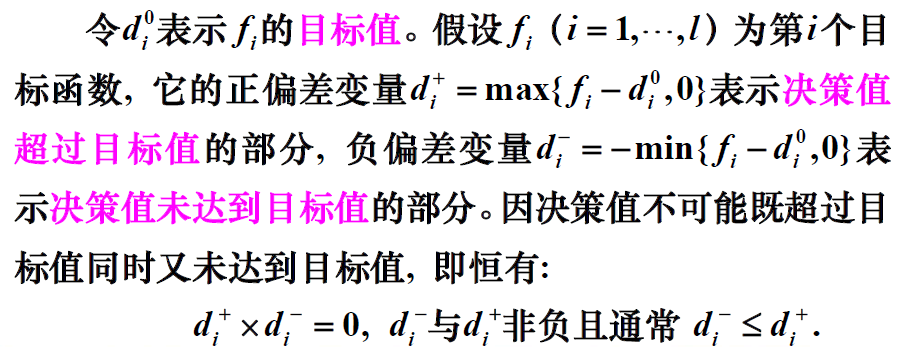绝对（刚性）约束和目标约束
绝对约束是指必须严格满足的等式约束和不等式约束,例如线性规划问题的所有约束条件。不能满足这些约束条件的解称为非可行解,所以它们是硬约束,钢性约束。
目标约束是目标规划特有的,可把约束右端项看作要追求的目标值。在达到此目标值时允许发生正或负偏差,因此在这些约束中加入正、负偏差变量,它们是软约束,柔性约束。
正负偏差变量的作用：可将绝对约束通过添加正、负偏差变量的方式转化为柔性约束。
例：
条件1：产品I的产量不大于产品II;
条件2：应尽可能充分利用设备, 但不希望加班;
条件3：应尽可能达到并超过计划利润指标56万元;
硬性约束：
x1<=x2;
x1+2x2<=10;
z=8x1+10*x2;
通过添加正负偏差变量转化为柔性约束：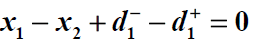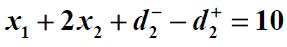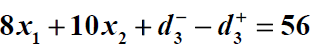优先因子（优先等级）与权系数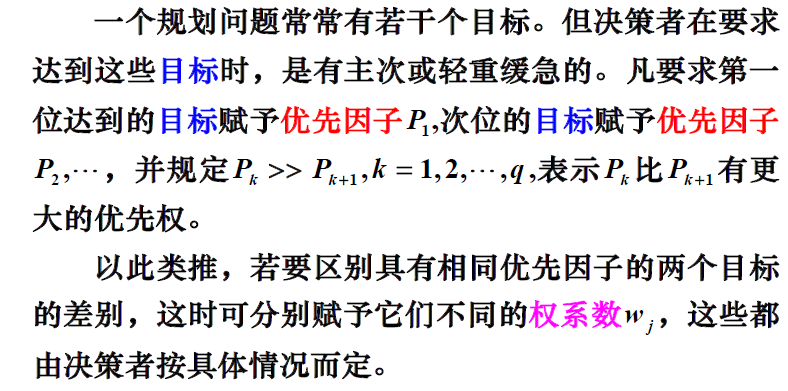一般而言，要求排在前面的优先考虑，优先级更高，优先因子更大。
目标规划的目标函数
与线性规划的目标函数构造不同,目标规划方法的目标函数（准则函数）是按各目标约束的正、负偏差变量,并赋于相应的优先因子和权系数而构造的。当每一目标值确定后，决策者的要求是尽可能缩小偏离目标值。因此目标规划的目标函数只能是所有偏差变量的加权和。其基本形式有三种。
(1)第i个目标要求恰好达到目标值，即正、负偏差变量都要尽可能地小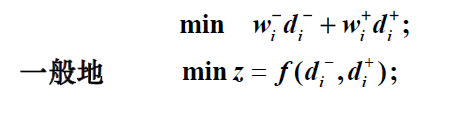(2)第i个目标要求不超过(不大于)目标值, 即允许达不到目标值，就是正偏差变量要尽可能地小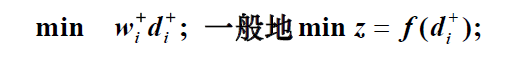(3)第i个目标要求超过(不小于)目标值, 即超过量不限, 但必须是负偏差变量要尽可能地小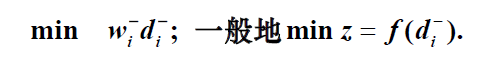目标规划的一般数学模型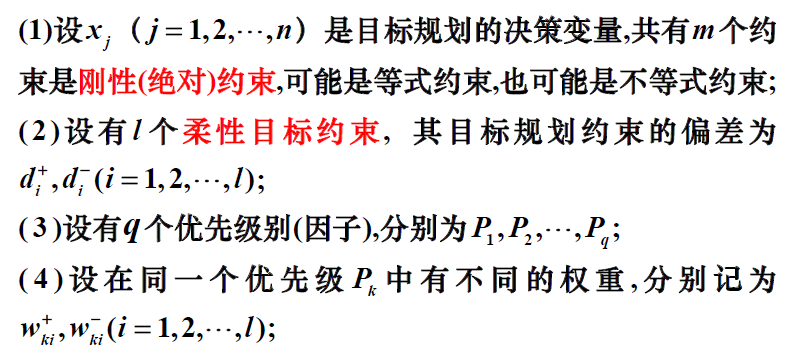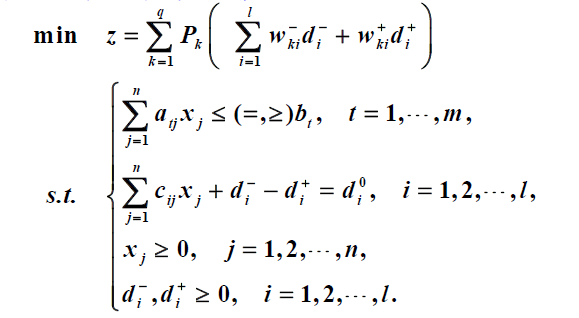建立目标规划的数学模型时,需要确定目标值、优先等级、权系数等,它们都具有一定的主观性和模糊性,可以用专家评定法给以量化。
目标规划的续贯算法
根据优先级的先后次序, 将目标规划问题分解成一系列的单目标规划问题, 然后再依次求解。
注意：求下一级目标, 之前的目标规划全部作为约束条件。

所有目标一个一个的求，求出前面的目标后，在后面目标的求解中将前面目标的结果作为刚性约束。
例：某企业生产甲、乙两种产品,需要用到A,B,C三种设备,关于产品的赢利与使用设备的工时及限制如表2 所示。问该企业应如何安排生产,才能达到下列目标。
（1）力求使利润指标不低于1500 元；
（2）考虑到市场需求,甲、乙两种产品的产量比应尽量保持1:2；
（3）设备A为贵重设备,严格禁止超时使用；
（4）设备C可以适当加班,但要控制；设备B既要求充分利用,又尽可能不加班。在重要性上,设备B是设备C的3 倍。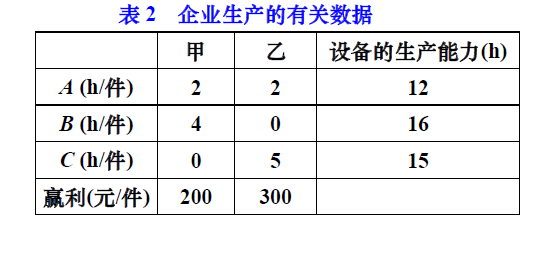解：由条件分析,得：
设备A是刚性约束, 其余是柔性约束;
首先, 最重要的指标是企业的利润, 因此将它的优先级列为第一级；
其次, 甲、乙两种产品的产量保持1:2的比例, 列为第二级；
再次, 设备B,C的工作时间要有所控制, 列为第三级;在第三级中, 设备B的重要性是设备C的三倍, 因此它们的权重不一样, 设备B前的系数是设备C前系数的3倍。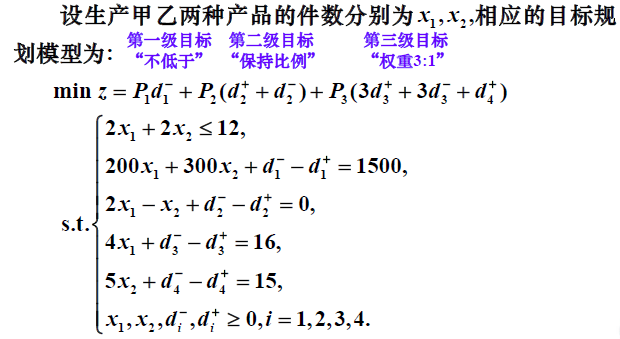多目标规划的Lingo解法
序贯算法中每个单目标问题都是一个线性规划问题, 可以使用
Lingo软件进行求解。
以上例为例，利用lingo求解如下：
第一级：
model:
sets:
variable/1,2/:x;
s_con_num/1..4/:g,dplus,dminus; !目标约束项数;
s_con(s_con_num,variable):c;
!dplus=d+,dminus=d-,目标约束系数c=c(i,j);
endsets
data:
g=1500 0 16 15;c=200 300 2 -1 4 0 0 5; !默认按行赋值;
enddata
min=dminus(1); !一级目标函数;
2*x(1)+2*x(2)<=12;
@for(s_con_num(i):@sum(variable(j):c(i,j)*x(j)+dminus(i)-dplus(i))=g(i););
@for(variable:@gin(x)); !变量取整约束;
end

第二级：
model:
sets:
variable/1,2/:x;
s_con_num/1..4/:g,dplus,dminus;
s_con(s_con_num,variable):c;
endsets
data:
g=1500 0 16 15;c=200 300 2 -1 4 0 0 5;
enddata
min=dplus(2)+dminus(2); !二级目标函数;
2*x(1)+2*x(2)<=12;
@for(s_con_num(i):@sum(variable(j):c(i,j)*x(j)+dminus(i)-dplus(i))=g(i););
dminus(1)=0; !一级目标约束;
@for(variable:@gin(x));
end

第三级：
model:
sets:
variable/1,2/:x;
s_con_num/1..4/:g,dplus,dminus;
s_con(s_con_num,variable):c;
endsets
data:
g=1500 0 16 15;c=200 300 2 -1 4 0 0 5;
enddata
min=3*dplus(3)+3*dminus(3)+dplus(4); !三级目标函数;
2*x(1)+2*x(2)<=12;
@for(s_con_num(i):@sum(variable(j):c(i,j)*x(j))+dminus(i)-dplus(i)=g(i););
dminus(1)=0;dplus(2)+dminus(2)=0; !一、二级目标约束;
@for(variable:@gin(x));
end

若三个阶段在一起求：
model:
sets:
level/1..3/:p,z,goal; !三个目标;
variable/1,2/:x;
s_con_num/1..4/:g,dplus,dminus;
s_con(s_con_num,variable):c;
h_con_num/1/:b;
h_con(h_con_num,variable):a;
obj(level,s_con_num)/1 1,2 2,3 3,3 4/:wplus,wminus; !权重;
endsets
data:
ctr=?;
goal=? ? 0;
b=12;
a=2 2;
g=1500 0 16 15;
c=200 300 2 -1 4 0 0 5;
wplus=0 1 3 1;
wminus=1 1 3 0;
enddata
min=@sum(level:p*z);
@for(level(i)|i#ne#ctr:p(i)=0);
@for(level(i):z(i)=@sum(obj(i,j):wplus(i,j)*dplus(j)+wminus(i,j)*dminus(j)););
@for(h_con_num(i):@sum(variable(j):a(i,j)*x(j))<=b(i););
@for(s_con_num(i):@sum(variable(j):c(i,j)*x(j))+dminus(i)-dplus(i)=g(i););
@for(level(i)|i#lt#@size(level):@bnd(0,z(i),goal(i)));
@for(variable:@gin(x));
end

多目标规划的matlab解法
通式总结：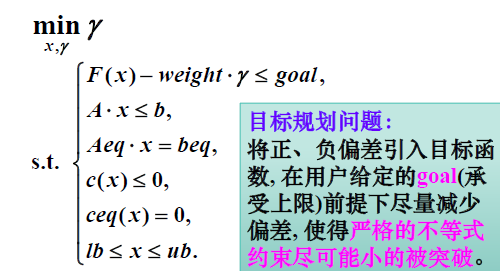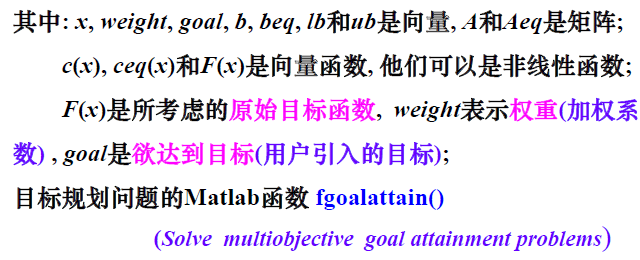fgoalattain()函数的用法：
x = fgoalattain(fun,x0,goal,weight)
x = fgoalattain(fun,x0,goal,weight,A,b)
x = fgoalattain(fun,x0,goal,weight,A,b,Aeq,beq)
x = fgoalattain(fun,x0,goal,weight,A,b,Aeq,beq,lb,ub)
x = fgoalattain(fun,x0,goal,weight,A,b,Aeq,beq,lb,ub,nonlcon)
x = fgoalattain(fun,x0,goal,weight,A,b,Aeq,beq,lb,ub,nonlcon,options)
x = fgoalattain(problem)
[x,fval] = fgoalattain(___)
[x,fval,attainfactor,exitflag,output] = fgoalattain(___)
[x,fval,attainfactor,exitflag,output,lambda] = fgoalattain(___)
例：求解多目标线性规划问题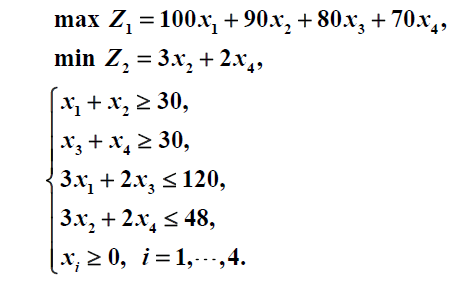（1）定义目标函数：
function F=Fun16_5(x)
F=[-100*x(1)-90*x(2)-80*x(3)-70*x(4);3*x(2)+2*x(4)];
end

（2）goal由两个目标函数分别求出目标值合并而成;weight由abs(goal)取定
a=[-1 -1 0 0;0 0 -1 -1;3 0 2 0;0 3 0 2]
b=[-30,-30,120,48].'
c1=-[100,90,80,70]
c2=[0,3,0,2]
[x1,goal1]=linprog(c1,a,b,[],[],zeros(4,1))
[x2,goal2]=linprog(c2,a,b,[],[],zeros(4,1))
goal=[goal1;goal2]
[x,fval]=fgoalattain('Fun16_5',rand(4,1),goal,abs(goal),a,b,[],[],zeros(4,1))



展开全文• 线性规划.非线性规划.多目标规划Matlab代码[比赛已经用过,保真好使]
• 里面包含了灰狼算法求解多目标规划的基本代码并，同时还有相关改进的代码与改进的参照论文，除此之外，还有用灰狼算法优化svm算法的代码
• 数学建模（3.9）多目标规划 理解 多目标规划跟一般的规划问题有所不同，多目标规划通常是要求学生做出满足各个优先度要求的最佳抉择。衡量出尽量满足所有需求而得出使得目标最优（如收益最大）的方案。 由于...
数学建模（3.9）多目标规划
理解
多目标规划跟一般的规划问题有所不同，多目标规划通常是要求学生做出满足各个优先度要求的最佳抉择。衡量出尽量满足所有需求而得出使得目标最优（如收益最大）的方案。
由于多目标规划跟线性规划完全不同，因此在此需要使用全新的解法。
正负偏差
为了将约束条件转换为等式，使得转换变成对偏差量的求解。在此引入d1,d1_，分别代表正负偏差变量。
d1=max{ fn-dn , 0 }表示决策值超过目标值的部分
d1_=-min{ f-dn , 0 }表示决策值未达到目标值的部分
前面的分段函数，是为了保证正负偏差变量不会出现负数情况
显然决策值只会要么多余目标值要么少于目标值，即b1,b1_中必定有一个为0
刚性约束和柔性约束
顾名思义，一定要满足的约束条件为刚性约束，尽量满足的约束条件为柔性约束条件。
其中刚性约束条件也可以不使用正负偏差变量代替。
优先等级
在随后的求解过程中，会出现达成目标的轻重缓急。在前的达成优先度会高于后者。
目标函数
（1）
要求尽可能接近（恰好达到目标值）
即要求正负偏差变量都尽可能的小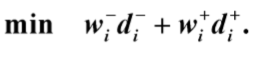表示为使负偏差变量与系数乘积+正偏差变量与系数乘积最小
（2）
要求不能超过目标值（例如不能超过预算），即允许达不到目标值，使得正偏差变量尽可能的小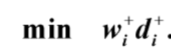表示为正偏差变量与系数乘积最小
（3）
要求能超过目标值，即超过量不限，但是负偏差尽可能要小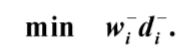表示为负偏差变量与系数乘积最小
实例

某单位领导在考虑本单位职工的升级调资方案时，要求相关部门遵守以下的规定：

（1）年工资总额不超过1500000元；
（2）每级的人数不超过定编规定的人数；
（3）II、III级的升级面尽可能达到现有人数的20%；
（4）III级不足编制的人数可录用新职工，又I级的职工中有10%的人要退休. 相关资料汇总于表2-1中，试为单位领导拟定一个满足要求的调资方案.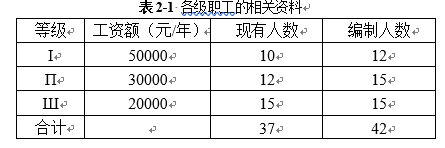求解思路
为了考虑选取最优的调资方案，需要考虑三个约束条件，显然前两个约束条件为刚性约束，而第三个约束条件为柔性约束。
分别建立目标约束
设由II晋升为I的人数为x1，由III晋升为II的人数为x2，招聘为III的人数为x3，dn_为未满误差，dn为过盈误差,n=1.2.3.4.5
年工资总额不超标
（1）
为保证调资后的年工资预算仍在指标范围内，有约束条件（2）
每一级的人数不超过定编规定的人数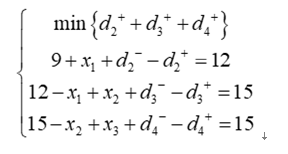（3）
II，III的升级面尽量达到现有人数的20%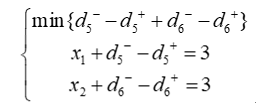最终得到目标规划的数学模型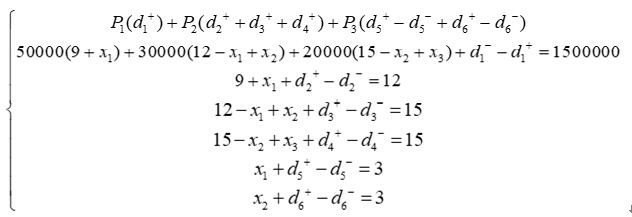通过lingo软件求解得第一级偏差和第二级偏差都为0，代入求得第三极偏差为-1
代入原模型解得
X1=2
X2=4
X3=6
lingo求解程序请见传送门：
https://blog.csdn.net/qq_43649786/article/details/98359558
更多内容请关注公众号：拾壹纪元展开全文• 论文研究-多年滚动开发用多目标规划型生态经济发展规划模型研究.pdf, 本文将待规划区域的国土按地貌特征进行分类, 以所得的各微地貌上适宜的优化型种、养殖业模式群为...
• 多目标规划的LINGO求解法 对于对目标问题的一种比较好的方法...